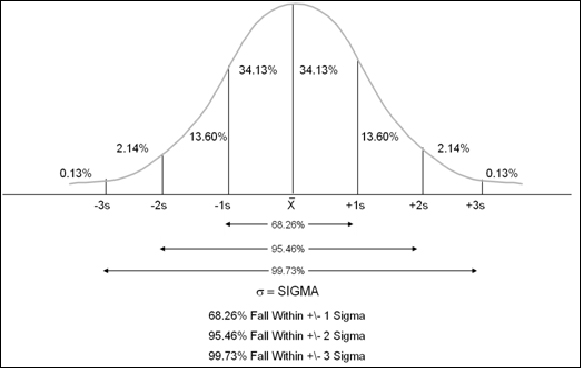# Python

Python has recently emerged as a preferred programming language for data analysis.

It has applications across data science pipelines that convert data into a usable format (such as pandas), analyzes it (such as NumPy), and extract useful conclusions from the data to represent it in a visually appealing manner (such as Matplotlib ,seaborn or Bokeh).

Python provides data visualization libraries that can help you easily access graphical representations.

Visualization is a critical component in exploratory data analysis, as well as presentations and applications.

## Dash Core Components

PictureDash ships with supercharged components for interactive web browser user interfaces. A core set of components, written and maintained by the Dash team, is available in the `dash-core-components` library.

The core components add to the html/javascript built in functions.

Take a quick look at many of the available input and output functionality including:

• Dropdown
• Slider
• RangeSlider
• Input
• Textarea
• Checklist
• Input Button
• DatePickerSingle

## Dash Daq

PictureDash is a web application framework that provides pure Python abstraction around HTML, CSS, and JavaScript.

Dash DAQ comprises a robust set of controls that make it simpler to integrate data acquisition and controls into your Dash applications.

The source is on GitHub at plotly/dash-daq.

Here is a simple example using most of the Dash Daq items.

## TkFrames_passed_vars

PictureTk example for class frame display and variable passing.

This is a simple button and Menu example of changing frames based on class definitions.

Variable get and pass between frames.

Scroll text message from frames.

## Python Pandas Descriptive Statistics

PictureStatistics can be of two types: descriptive and inferential.

Descriptive statistics describe characteristics about a population or a sample of data.

Inferential statistics involves drawing inferences about the characteristics of a population from a sample of data.

Descriptive statistics assumes a normal distribution:Also known as a normal curve, the characteristics of normal distribution are as follows:

Most data distributions can be characterized by two different measures:

• Central tendency, which measures using the mean, mode, and median

• Dispersion, which can be measured using range and standard deviation

Here is a simple python app taking advantage of pandas, numpy, and , matplotlib .

Python Pandas - Descriptive Statistics

Python Pandas Functions & Description
Function  Description
count()   Number of non-null observations
sum()       Sum of values
mean()       Mean of Values
median()  Median of Values
mode()       Mode of values
std()       Standard Deviation of the Values
min()       Minimum Value
max()       Maximum Value
abs()       Absolute Value
prod()       Product of Values
cumsum()  Cumulative Sum
cumprod() Cumulative Product

FYI pandas.describe() includes count, mean, min, max, std, and 25%,50%,& 75% quartiles

## Polynomial Regression

PictureCalibrating a sensor with input and output values for automation tends be very common for translating a sensor value into a real world value.

Most but not all regression fits are linear.

If a polynomial equation fit is required, this will help.

Taking advantage of Python modules pandas,sklearn, & matplotlib makes it easy to do.

Here is a simple application with the source code and an example data CSV file.

## Linear Regression

PictureCalibrating a sensor with input and output values for automation tends be very common for translating a sensor value into a real world value.

Taking advantage of Python modules pandas,sklearn, & matplotlib makes it easy to do.

Here is a simple application with the source code and an example data CSV file.

## Pandas Pivot Table

PicturePivot tables are useful with a long list of data in a spreadsheet and presenting the summarized data as a function of one or more columns.

( ie the total sales \$ per seller, or the total sales \$ of each product or the total sales per week. . . )

If you are familiar with spreadsheets, you are probably thinking about using a pivot table to get the average of the variables for each cluster. In SQL, you would have probably used a GROUP BY statement. If you are not familiar with either of these, you may think of grouping each cluster together and then calculating the average for each of them.

To create a pivot table similar to a spreadsheets, we will be using the pivot_table() method from pandas.

If you are not familiar with using a pivot table in a spreadsheet, you can see the finished tables in the xlsx file using Libre Calc or MS Excel.

The python pandas pivot tables are the same.

## TK_Frames_V3_Everything

PictureThis Python Tkinter Multi frames GUI is based on TK_Frames_V3.txt at:

In addition to the base frames functionality, most widget examples are include with get() code examples.

Here you can see how the sequential functionality of Python can integrate into the event oriented GUI.

## TK_Frames_V2_Everything

PictureThis Python Tkinter Multi frames GUI is based on TK_Frames_V2.txt at:

In addition to the base frames functionality, most widget examples are include with get() code examples.

Here you can see how the sequential functionality of Python can integrate into the event oriented GUI.

## TK_Frames_V1_Everything

PictureThis Python Tkinter Multi frames GUI is based on TK_Frames_V1.txt at: